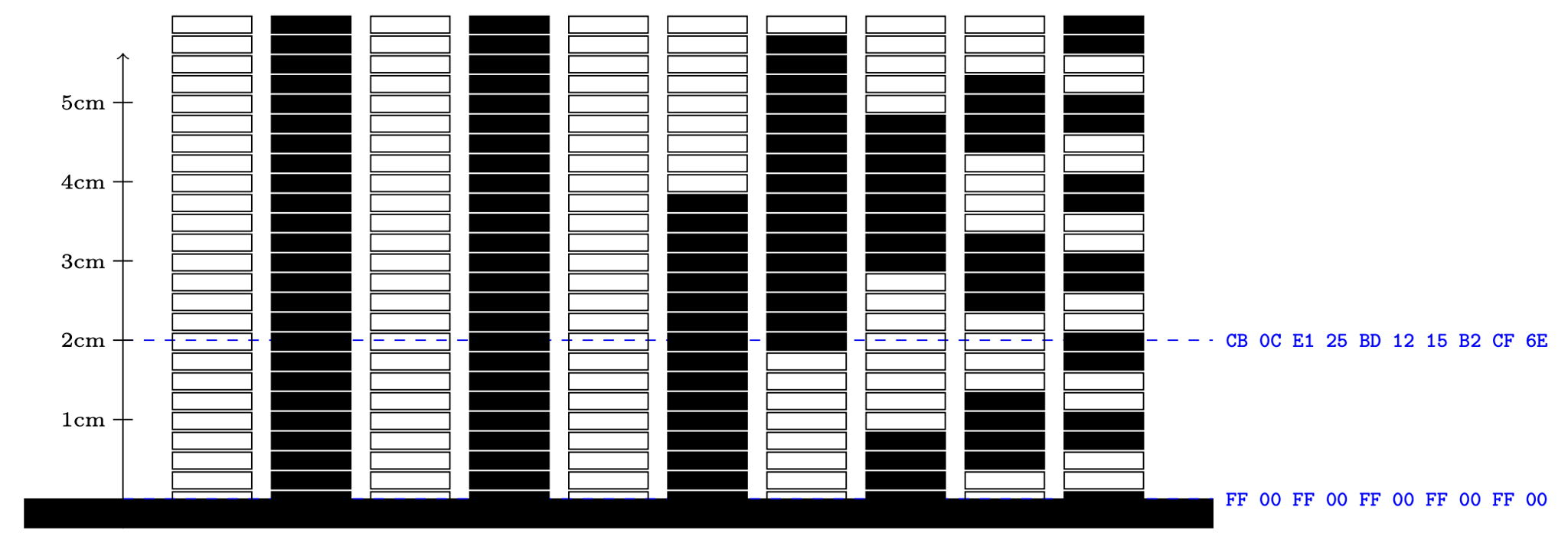시간 제한 메모리 제한 제출 정답 맞은 사람 정답 비율
1 초 512 MB 1 1 1 100.000%

## 문제

A stadiometer is a medical tool used to measure a person's height. In the olden days, stadiometers were just made out of a head piece rolling on a graduated ruler. But this old-fashioned technology easily led to inaccurate readings because the wheel can become decalibrated in the blink of an eye, especially with restless children.

Modern stadiometers use optical readings. They are usually able to measure your height with a precision of $0.25$ cm. The way they work is that a special pattern of height $0.25$ cm is printed on the wall, a laser sends a beam of light right above your head, and a photo-sensor detects the pattern that is exactly behind. There are $1024$ patterns so that the device can return 1024 measurement results from 0 cm to 255.75 cm.

The International Colours & Patterns Committee has designed an open standard with the follwing rules. Each pattern consists of 10 horizontally arranged rectangles that are either black or white. From one pattern to the next, only one rectangle changes which is useful to avoid errors with the optical reading. Obviously, if the photo-sensor is positioned at the middle of two patterns and averages the readings for the two patterns, then only one rectangle can be affected and the measure is only off by $0.25$ cm.

The 1024 patterns are generated in the following way:

•  In the first column, the $512$ bottom rectangles are white and the $512$  top rectangles are black.
• For $2\le k \le 10$, the $k$th column is divided into  $2^{k-2}$ blocks of $2^{12-k}$ rectangles:
• the $2^{10-k}$ rectangles at the bottom and at the top of the block are white if $k$ is odd and black if $k$ is even;
• the $2^{11-k}$ rectangles in the middle of the block are black if $k$ is odd and white if $k$ is even.The optical reader reports 10 bytes (integer values between 0 and 255) which stand for 256 shades of grey. Any number between 0 and 127 is interpreted as a black rectangle whereas any number between 128 and 255 is interpreted as a white rectangle.

Given the 10 integers reported by the optical reader, can you find the correct height of the person according to the I.C.P.C. standard?

## 입력

The input file consists of multiple test cases. The first line of the input file consists of a single integer indicating the number of test cases. Each test case follows and consists of a single line consisting of ten pairs of characters separated by single spaces. Each character pair stands for a number between 0 and 255 written in hexadecimal notation.

## 출력

For each test case in the input, your program should produce one line consisting of one float with exactly two digits after the decimal point that indicates the height of the person that has been measured.

## 예제 입력 1

2
FF 00 FF 00 FF 00 FF 00 FF 00
CB 0C E1 25 BD 12 15 B2 CF 6E


## 예제 출력 1

0.00
2.00